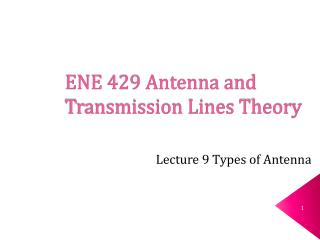Download PresentationENE 429 Antenna and Transmission Lines Theory

Loading in 2 Seconds...

# ENE 429 Antenna and Transmission Lines Theory - PowerPoint PPT Presentation

ENE 429 Antenna and Transmission Lines Theory. Lecture 9 Types of Antenna. Review (1). Antenna is a structure designed for radiating and receiving EM energy in a prescribed mannerI am the owner, or an agent authorized to act on behalf of the owner, of the copyrighted work described.
Download Presentation## ENE 429 Antenna and Transmission Lines Theory

An Image/Link below is provided (as is) to download presentation

Download Policy: Content on the Website is provided to you AS IS for your information and personal use and may not be sold / licensed / shared on other websites without getting consent from its author.While downloading, if for some reason you are not able to download a presentation, the publisher may have deleted the file from their server.

- - - - - - - - - - - - - - - - - - - - - - - - - - E N D - - - - - - - - - - - - - - - - - - - - - - - - - -
Presentation Transcript
1. ENE 429 Antenna and Transmission Lines Theory Lecture 9 Types of Antenna

2. Review (1) • Antenna is a structure designed for radiating and receiving EM energy in a prescribed manner • Far field region ( the distance where the receiving antenna is located far enough for the transmitter to appear as a point source) • The shape or pattern of the radiated field is independent of r in the far field. • Normalized power function or normalized radiation intensity

3. Review (2) • Directivity is the overall ability of an antenna to direct radiated power in a given direction. • An antenna’s pattern solid angle: • Total radiated power can be written as • Antenna efficiency e is measured as

4. Radiation characteristics • If the current distribution of a radiating element is known, we can calculate radiated fields. • In general, the analysis of the radiation characteristics of an antenna follows the three steps below: • Determine the vector magnetic potential from known of assumed current on the antenna. • Find the magnetic field intensity from . • Find the electric field intensity from .

5. Vector magnetic potential From the point form of Gauss’s law for magnetic field, Define therefore we can express as where Jd= current density at the point source (driving point) R = distance from the point source to the observation point (m)

6. Electric and magnetic fields can be determined. • From here we can determine , then find in free space. • We can then find the electric field from • The time-averaged radiated power is The subscript “0” represents the observation point. W/m2.

7. Types of antennas • Hertzian dipole (electric dipole) • Small loop antenna (magnetic dipole) • Dipole antenna

8. Hertzian dipole (1) • A short line of current that is short compared to the operating wavelength. This thin, conducting wire of a length dl carries a time-harmonic current A and in a phasor form A.

9. Hertzian dipole (2) The current density at the source seen by the observation point is A differential volume of this current element is dV = Sdz.

10. Hertzian dipole (3) Therefore Then Where at the observation point. For short dipole, R  r, thus we can write Conversion into the spherical coordinate gives

11. Hertzian dipole (4) Therefore We can then calculate for

12. Hertzian dipole (5) Multiply 2 to both nominator and denominator, so we have We are interested in the fields at distances very far from the antenna, which is in the region where or

13. Hertzian dipole (6) Under a far-field condition, we could neglect and Then and Finally, W/m2.

14. Hertzian dipole (7) Since the current along the short Hertzian dipole is uniform, we refer the power dissipated in the radial distance Rradto I, m. or

15. Ex2 Find the radiation efficiency of an isolated Hertzian dipole made of a metal wire of radius a, length d, and conductivity .

16. Ex1 Let a Hertzian dipole of the length /100 be given the current A, find • Pmax at r = 100 m

17. b) What is the time-averaged power density at P (100, /4, /2)? c) Radiation resistance

18. The small loop antenna (magnetic dipole) Assume a <<  A complicate derivation brings to If the loop contains N-loop coil then S = Na2

19. Dipole antennas • Longer than Hertizian dipole therefore they can generate higher radiation resistance and efficiency. Divide the dipole into small elements of Hertzian dipole. Then find and . Figure of dipole

20. Dipole antenna radiation regime

21. Field derivations (Far field) (1) The current on the two halves are Symmetrical and go to zero at the ends. We can write Where Assume  = 0 for simplicity.

22. Field derivations (Far field) (2) From In far field but since small differences can be critical.

23. Field derivations (Far field) (3) We can write

24. Field derivations (Far field) (3) From In our case

25. Antenna properties • Find Pn(), calculate F() over the full range of  for length L in terms of wavelength then find Fmax(this step requires Matlab) • Find p • Dmax (Directivity) 4. Rrad

26. Antenna Radiation Pattern for different antenna lenghts • Link to Matlab file

27. Half-wave dipole (most popular antenna) (1)

28. Half-wave dipole (most popular antenna) (2) Using Matlab, we get p = 7.658 Dmax = 1.64 Rrad = 73.2  This is much higher than that of the Hertzian dipole.